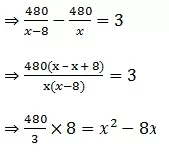# A train travels a distance of 480 km at a uniform speed

A train travels a distance of 480 km at a uniform speed.

Distance travelled by the train = 480 km
Let the speed of the train be x kmph
Time taken for the journey = 480/x
Given speed is decreased by 8 kmph
Hence the new speed of train = (x – 8) kmph
Time taken for the journey = 480/(x – 8)�1280 = x2�– 8x
�x2�– 8x – 1280 = 0
On solving we get x = 40
Thus the speed of train is 40 kmph